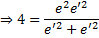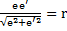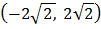# If a variable line has its intercepts on the coordinate axeswhereare the eccentricities of a hyperbola and its conjugate hyperbola, then the line always touches the circle, wherea) 1 b) 2 c) 3 d) cannot be decided

## Question ID - 55844 :- If a variable line has its intercepts on the coordinate axeswhereare the eccentricities of a hyperbola and its conjugate hyperbola, then the line always touches the circle, wherea) 1 b) 2 c) 3 d) cannot be decided

3537

(b)

Sinceandare eccentricities of a hyperbola and its conjugate hyperbola, thereforeThe line passing through the pointsandisIt is tangents to the circleHence,Next Question :
 If two chords drawn from the point(4, 4) to the parabolaare bisected by linethe interval in which lies is a)b)c)d) None of these